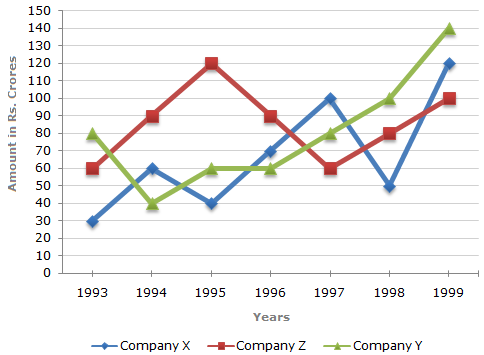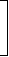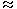# Data Interpretation - Line Charts

## Why Data Interpretation Line Charts?

In this section you can learn and practice Data Interpretation Questions based on "Line Charts" and improve your skills in order to face the interview, competitive examination and various entrance test (CAT, GATE, GRE, MAT, Bank Exam, Railway Exam etc.) with full confidence.

## Where can I get Data Interpretation Line Charts questions and answers with explanation?

IndiaBIX provides you lots of fully solved Data Interpretation (Line Charts) questions and answers with Explanation. Solved examples with detailed answer description, explanation are given and it would be easy to understand. All students, freshers can download Data Interpretation Line Charts quiz questions with answers as PDF files and eBooks.

## Where can I get Data Interpretation Line Charts Interview Questions and Answers (objective type, multiple choice)?

Here you can find objective type Data Interpretation Line Charts questions and answers for interview and entrance examination. Multiple choice and true or false type questions are also provided.

## How to solve Data Interpretation Line Charts problems?

You can easily solve all kind of Data Interpretation questions based on Line Charts by practicing the objective type exercises given below, also get shortcut methods to solve Data Interpretation Line Charts problems.

### Exercise :: Line Charts - Line Chart 1

Study the following line graph and answer the questions.

Exports from Three Companies Over the Years (in Rs. crore)1.

For which of the following pairs of years the total exports from the three Companies together are equal?

 A. 1995 and 1998 B. 1996 and 1998 C. 1997 and 1998 D. 1995 and 1996

Explanation:

Total exports of the three Companies X, Y and Z together, during various years are:

In 1993 = Rs. (30 + 80 + 60) crores = Rs. 170 crores.

In 1994 = Rs. (60 + 40 + 90) crores = Rs. 190 crores.

In 1995 = Rs. (40 + 60 + 120) crores = Rs. 220 crores.

In 1996 = Rs. (70 + 60 + 90) crores = Rs. 220 crores.

In 1997 = Rs. (100 + 80 + 60) crores = Rs. 240 crores.

In 1998 = Rs. (50 + 100 + 80) crores = Rs. 230 crores.

In 1999 = Rs. (120 + 140 + 100) crores = Rs. 360 crores.

Clearly, the total exports of the three Companies X, Y and Z together are same during the years 1995 and 1996.

2.

Average annual exports during the given period for Company Y is approximately what percent of the average annual exports for Company Z?

 A. 87.12% B. 89.64% C. 91.21% D. 93.33%

Explanation:

Analysis of the graph: From the graph it is clear that

1. The amount of exports of Company X (in crore Rs.) in the years 1993, 1994, 1995, 1996, 1997, 1998 and 1999 are 30, 60, 40, 70, 100, 50 and 120 respectively.

2. The amount of exports of Company Y (in crore Rs.) in the years 1993, 1994, 1995, 1996, 1997, 1998 and 1999 are 80, 40, 60, 60, 80, 100 and 140 respectively.

3. The amount of exports of Company Z (in crore Rs.) in the years 1993, 1994, 1995, 1996, 1997, 1998 and 1999 are 60, 90,, 120, 90, 60, 80 and 100 respectively.

Average annual exports (in Rs. crore) of Company Y during the given period

 = 1 x (80 + 40 + 60 + 60 + 80 + 100 + 140) = 560 = 80. 7 7

Average annual exports (in Rs. crore) of Company Z during the given period

 = 1 x (60 + 90 + 120 + 90 + 60 + 80 + 100) =600. 7 7Required percentage =80 x 100%93.33%.6007

3.

In which year was the difference between the exports from Companies X and Y the minimum?

 A. 1994 B. 1995 C. 1996 D. 1997

Explanation:

The difference between the exports from the Companies X and Y during the various years are:

In 1993 = Rs. (80 - 30) crores = Rs. 50 crores.

In 1994 = Rs. (60 - 40) crores = Rs. 20 crores.

In 1995 = Rs. (60 - 40) crores = Rs. 20 crores.

In 1996 = Rs. (70 - 60) crores = Rs. 10 crores.

In 1997 = Rs. (100 - 80) crores = Rs. 20 crores.

In 1998 = Rs. (100 - 50) crores = Rs. 50 crores.

In 1999 = Rs. (140 - 120) crores = Rs. 20 crores.

Clearly, the difference is minimum in the year 1996.

4.

What was the difference between the average exports of the three Companies in 1993 and the average exports in 1998?

 A. Rs. 15.33 crores B. Rs. 18.67 crores C. Rs. 20 crores D. Rs. 22.17 crores

Explanation:

Average exports of the three Companies X, Y and Z in 1993

 = Rs.1 x (30 + 80 + 60)crores = Rs.170crores. 3 3

Average exports of the three Companies X, Y and Z in 1998

 = Rs.1 x (50 + 100 + 80)crores = Rs.230crores. 3 3

Difference
 = Rs.230-170crores 3 3
 = Rs.60crores 3
= Rs. 20 crores.

5.

In how many of the given years, were the exports from Company Z more than the average annual exports over the given years?

 A. 2 B. 3 C. 4 D. 5

Explanation:

Average annual exports of Company Z during the given period

 = 1 x (60 + 90 + 120 + 90 + 60 + 80 + 100) 7

 = Rs.600crores 7

= Rs. 85.71 crores.

From the analysis of graph the exports of Company Z are more than the average annual exports of Company Z (i.e., Rs. 85.71 crores) during the years 1994, 1995, 1996 and 1999, i.e., during 4 of the given years.

#### Current Affairs 2019

Interview Questions and Answers